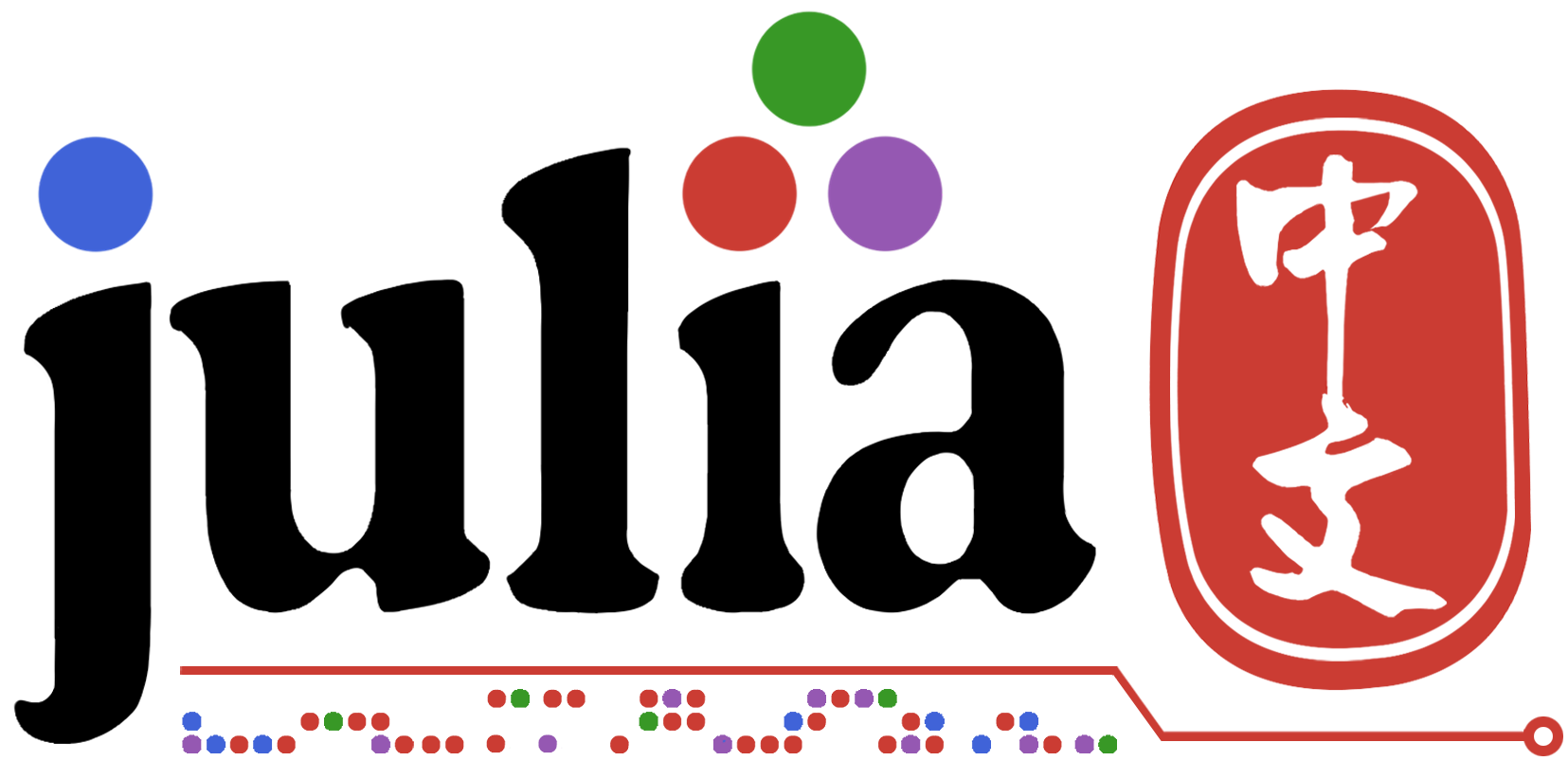# 关于 struct, Vector 在函数中使用的疑惑

``````mutable struct DataSets
length
height
weight
end

function fun1(x::DataSets)
y = x.length
x.height = fun(y)
end

x = DataSets(1.0, 1.0, 1.0)
fun1(x)
``````

``````function fun2(x::DataSets)
y = x.length
height = fun(y)
return DataSets(x.length, height, x.weight)
end

x = DataSets(1.0, 1.0, 1.0)
fun2(x)
``````

1. 我给出的两种方案，哪种是 `Julia` 编码规范更推荐的做法？或者说还有更优的解决方案？
2. 对于 `Julia``Vector` 修改，我也有同样的疑惑。是修改传入的 `Vector` 好？还是返回一个新 `Vector` 更好？

———————————————————————————————————————

1 个赞

Vector/Array 一般来说都不小，默认就是可变的，重新分配成本较大。julia 里有很多原地修改的函数，以 `!` 结尾，就是为了避免内存分配，影响性能。推荐的也是原地修改。实际上原地修改等价于 C 里传入指针。

``````struct DataSets{T}
length::T
height::T
weight::T
end
Base.copy(x::DataSets) = DataSets(x.length, x.height, x.weight)

using BenchmarkTools
x = DataSets(1, 1, 1)
@btime copy(\$x) # 0.046 ns (0 allocations: 0 bytes)
``````

``````struct Datasets{AT<:AbstractArray}
x::AT
y::AT
end
``````

``````mutable struct MPoint{T}
x::T
y::T
end

struct Point{T}
x::T
y::T
end

f(p) = p.x^2 + p.y^2

X = reshape([Point(rand(), rand()) for _ in 1:10000], 100, 100);

mX = reshape([MPoint(rand(), rand()) for _ in 1:10000], 100, 100);

@btime mapreduce(f, +, \$X); #2.443 μs (0 allocations: 0 bytes)

@btime mapreduce(f, +, \$mX); # 9.682 μs (0 allocations: 0 bytes)
``````
1 个赞

``````struct MyWrapper
data
end

X = MyWrapper(rand(4, 4))
X.data = zeros(4, 4) # 这里会报错：因为 X 是不可变的，所以不能修改 data 所指向的地址
fill!(X.data, 0) # 但是 X.data 是可变的，所以可以修改 data 所指向的地址的内容
``````
1 个赞